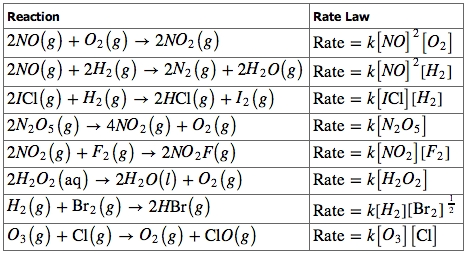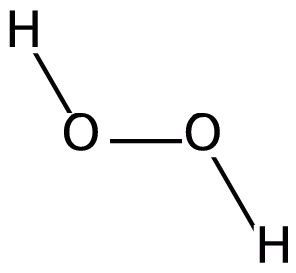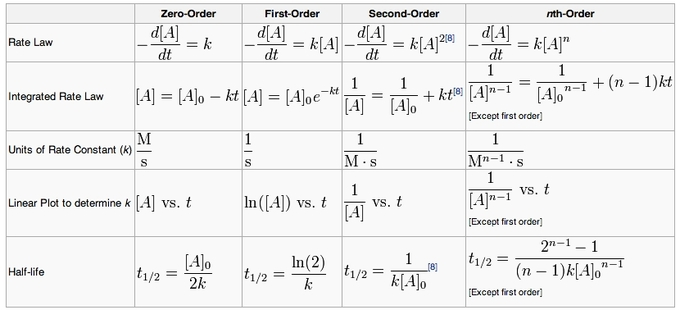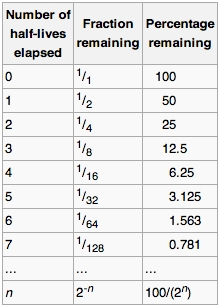## The Rate Law

The rate law for a chemical reaction relates the reaction rate with the concentrations or partial pressures of the reactants.

### Learning Objectives

Produce rate equations for elementary reactions

### Key Takeaways

#### Key Points

• For a generic reaction $\text{aA} + \text{bB} \rightarrow \text{C}$ with no intermediate steps in its reaction mechanism (that is, an elementary reaction), the rate is given by: $\text{r}=\text{k}[\text{A}]^{\text{x}}[\text{B}]^{\text{y}}$.
• For elementary reactions, the rate equation can be derived from first principles using collision theory.
• The rate equation of a reaction with a multi-step mechanism cannot, in general, be deduced from the stoichiometric coefficients of the overall reaction; it must be determined experimentally.

#### Key Terms

• Rate law: An equation relating the rate of a chemical reaction to the concentrations or partial pressures of the reactants.

The rate law for a chemical reaction is an equation that relates the reaction rate with the concentrations or partial pressures of the reactants. For the general reaction$\text{aA} + \text{bB} \rightarrow \text{C}$ with no intermediate steps in its reaction mechanism, meaning that it is an elementary reaction, the rate law is given by:

$\text{r}=\text{k}[\text{A}]^{\text{x}}[\text{B}]^{\text{y}}$

In this equation, [A] and [B] express the concentrations of A and B, respectively, in units of moles per liter. The exponents x and y vary for each reaction, and they must be determined experimentally; they are not related to the stoichiometric coefficients of the chemical equation. Lastly, k is known as the rate constant of the reaction. The value of this coefficient k will vary with conditions that affect reaction rate, such as temperature, pressure, surface area, etc. A smaller rate constant indicates a slower reaction, while a larger rate constant indicates a faster reaction.Rate laws for various reactions: A variety of reaction orders are observed. Note that the reaction order is unrelated to the stoichiometry of the reactions; it must be determined experimentally.

### Reaction Order

To reiterate, the exponents x and y are not derived from the balanced chemical equation, and the rate law of a reaction must be determined experimentally. These exponents may be either integers or fractions, and the sum of these exponents is known as the overall reaction order. A reaction can also be described in terms of the order of each reactant. For example, the rate law $\text{Rate}=\text{k}[\text{NO}]^2[\text{O}_2]$ describes a reaction which is second-order in nitric oxide, first-order in oxygen, and third-order overall. This is because the value of x is 2, and the value of y is 1, and 2+1=3.

### Example 1

A certain rate law is given as $\text{Rate}=\text{k}[\text{H}_2][\text{Br}_2]^\frac{1}{2}$. What is the reaction order?

$\text{x}=1,\;\text{y}=\frac{1}{2}$

$\text{reaction}\;\text{order}=\text{x}+\text{y}=1+\frac{1}{2}=\frac{3}{2}$

The reaction is first-order in hydrogen, one-half-order in bromine, and $\frac{3}{2}$-order overall.

### Example 2

The reaction between nitric oxide and ozone, $\text{NO}(\text{g}) + \text{O}_3(\text{g})\rightarrow \text{NO}_2(\text{g}) + \text{O}_2(\text{g})$, is first order in both nitric oxide and ozone. The rate law equation for this reaction is: $\text{Rate} = \text{k}[\text{NO}]^{1}[O_{3}]^{1}$. The overall order of the reaction is 1 + 1 = 2.

Rate of reactions tutorial: Paul Andersen defines the rate of a reaction as the number of reactants that are consumed during a given period of time. The rate of the reaction can be affected by the type of reaction as well as concentration, pressure, temperature and surface area.

## First-Order Reactions

A first-order reaction depends on the concentration of one reactant, and the rate law is: $\text{r}=-\frac{\text{dA}}{\text{dt}}=\text{k}[\text{A}]$.

### Learning Objectives

Design initial rate experiments to determine order of reaction with respect to individual reactants

### Key Takeaways

#### Key Points

• k is the first-order rate constant, which has units of 1/s.
• The method of determining the order of a reaction is known as the method of initial rates.
• The overall order of a reaction is the sum of all the exponents of the concentration terms in the rate equation.

#### Key Terms

• first-order reaction: A reaction that depends on the concentration of only one reactant (a unimolecular reaction). Other reactants can be present, but each will be zero-order.

A first-order reaction depends on the concentration of only one reactant. As such, a first-order reaction is sometimes referred to as a unimolecular reaction. While other reactants can be present, each will be zero-order, since the concentrations of these reactants do not affect the rate. Thus, the rate law for an elementary reaction that is first order with respect to a reactant A is given by:

$\text{r} = -\frac{\text{d}[\text{A}]}{\text{dt}} = \text{k}[\text{A}]$

As usual, k is the rate constant, and must have units of concentration/time; in this case it has units of 1/s.Hydrogen peroxide: The decomposition of hydrogen peroxide to form oxygen and hydrogen is a first-order reaction.

### Using the Method of Initial Rates to Determine Reaction Order Experimentally

$2\;\text{N}_{2}\text{O}_{5}(\text{g})\rightarrow 4\;\text{NO}_{2}(\text{g})+\text{O}_{2}(\text{g})$

The balanced chemical equation for the decomposition of dinitrogen pentoxide is given above. Since there is only one reactant, the rate law for this reaction has the general form:

$\text{Rate}= \text{k}[\text{N}_{2}\text{O}_{5}]^{\text{m}}$

In order to determine the overall order of the reaction, we need to determine the value of the exponent m. To do this, we can measure an initial concentration of N2O5 in a flask, and record the rate at which the N2O5 decomposes. We can then run the reaction a second time, but with a different initial concentration of N2O5. We then measure the new rate at which the N2O5 decomposes. By comparing these rates, it is possible for us to find the order of the decomposition reaction.

### Example

Let’s say that at 25 °C, we observe that the rate of decomposition of N2O5 is 1.4×10-3 M/s when the initial concentration of N2O5 is 0.020 M. Then, let’s say that we run the experiment again at the same temperature, but this time we begin with a different concentration of N2O5 , which is 0.010 M. On this second trial, we observe that the rate of decomposition of N2O5 is 7.0×10-4 M/s. We can now set up a ratio of the first rate to the second rate:

$\frac{\text{Rate}_1}{\text{Rate}_2}=\frac{\text{k}[\text{N}_2\text{O}_5]_{\text{i}1}^{\text{m}}}{\text{k}[\text{N}_{2}\text{O}_{5}]_{\text{i}2}^{\text{m}}}$

$\frac{1.4\times 10^{-3}}{7.0\times 10^{-4}}=\frac{\text{k}(0.020)^{\text{m}}}{\text{k}(0.010)^{\text{m}}}$

Notice that the left side of the equation is simply equal to 2, and that the rate constants cancel on the right side of the equation. Everything simplifies to:

$2.0=2.0^\text{m}$

Clearly, then, m=1, and the decomposition is a first-order reaction.

### Determining the Rate Constant k

Once we have determined the order of the reaction, we can go back and plug in one set of our initial values and solve for k. We find that:

$\text{rate}=\text{k}[\text{N}_2\text{O}_5]^1=\text{k}[\text{N}_2\text{O}_5]$

Substituting in our first set of values, we have

$1.4\times 10^{-3}=\text{k}(0.020)$

$\text{k}=0.070\;\text{s}^{-1}$

## Second-Order Reactions

A second-order reaction is second-order in only one reactant, or first-order in two reactants.

### Learning Objectives

Manipulate experimentally determined second-order rate law equations to obtain rate constants

### Key Takeaways

#### Key Points

• A second-order reaction will depend on the concentration (s) of one second-order reactant or two first-order reactants.
• To determine the order of a reaction with respect to each reactant, we use the method of initial rates.
• When applying the method of initial rates to a reaction involving two reactants, A and B, it is necessary to conduct two trials in which the concentration of A is held constant, and B changes, as well as two trials in which the concentration of B is held constant, and A changes.

#### Key Terms

• second-order reaction: A reaction that depends on the concentration(s) of one second-order reactant or two first-order reactants.
• reaction mechanism: The step-by-step sequence of elementary transformations by which overall chemical change occurs.

A reaction is said to be second-order when the overall order is two. For a reaction with the general form $\text{aA}+\text{bB}\rightarrow \text{C}$, the reaction can be second order in two possible ways. It can be second-order in either A or B, or first-order in both A and B. If the reaction were second-order in either reactant, it would lead to the following rate laws:

$\text{rate}=\text{k}[\text{A}]^2$

or

$\text{rate}=\text{k}[\text{B}]^2$

The second scenario, in which the reaction is first-order in both A and B, would yield the following rate law:

$\text{rate}=\text{k}[\text{A}][\text{B}]$

### Applying the Method of Initial Rates to Second-Order Reactions

Consider the following set of data:Rates and initial concentrations for A and B: A table showing data for three trials measuring the various rates of reaction as the initial concentrations of A and B are changed.

If we are interested in determining the order of the reaction with respect to A and B, we apply the method of initial rates.

### Determining Reaction Order in A

In order to determine the reaction order for A, we can set up our first equation as follows:

$\frac{\text{r}_1}{\text{r}_2}=\frac{\text{k}[\text{A}]_1^\text{x}[\text{B}]_1^\text{y}}{\text{k}[\text{A}]_2^\text{x}[\text{B}]_2^\text{y}}$

$\frac{5.46}{12.28}=\frac{\text{k}(0.200)^\text{x}(0.200)^\text{y}}{\text{k}(0.300)^\text{x}(0.200)^\text{y}}$

Note that on the right side of the equation, both the rate constant k and the term $(0.200)^\text{y}$ cancel. This was done intentionally, because in order to determine the reaction order in A, we need to choose two experimental trials in which the initial concentration of A changes, but the initial concentration of B is constant, so that the concentration of B cancels. Our equation simplifies to:

$\frac{5.46}{12.28}=\frac{(0.200)^\text{x}}{(0.300)^\text{x}}$

$0.444=\left(\frac{2}{3}\right)^\text{x}$

$\text{ln}(0.444)=\text{x}\cdot ln\left(\frac{2}{3}\right)$

$\text{x}\approx 2$

Therefore, the reaction is second-order in A.

### Determining Reaction Order in B

Next, we need to determine the reaction order for B. We do this by picking two trials in which the concentration of B changes, but the concentration of A does not. Trials 1 and 3 will do this for us, and we set up our ratios as follows:

$\frac{\text{r}_1}{\text{r}_3}=\frac{\text{k}[\text{A}]_1^2[\text{B}]_1^\text{y}}{\text{k}[\text{A}]_3^2[\text{B}]_3^\text{y}}$

$\frac{5.46}{5.42}=\frac{\text{k}(0.200)^2(0.200)^\text{y}}{\text{k}(0.200)^2(0.400)^y}$

Note that both k and the concentrations of A cancel. Also, $\frac{5.46}{5.42}\approx 1$, so everything simplifies to:

$1=\frac{(0.200)^\text{y}}{(0.400)^\text{y}}$

$1=\left(\frac{1}{2}\right)^\text{y}$

$\text{y}=0$

Therefore, the reaction is zero-order in B.

### Overall Reaction Order

We have determined that the reaction is second-order in A, and zero-order in B. Therefore, the overall order for the reaction is second-order $(2+0=2)$, and the rate law will be:

$\text{rate}=\text{k}[\text{A}]^2$

Initial rate method of kinetics: Using the method of initial rates to determine reaction order.

## Zero-Order Reactions

A zero-order reaction has a constant rate that is independent of the concentration of the reactant(s); the rate law is simply $\text{rate}=\text{k}$.

### Learning Objectives

Use graphs of zero-order rate equations to obtain the rate constant and the initial concentration data

### Key Takeaways

#### Key Points

• For a zero-order reaction, increasing the concentration of the reacting species will not speed up the rate of the reaction.
• Zero-order reactions are typically found when a material that is required for the reaction to proceed, such as a surface or a catalyst, is saturated by the reactants.
• A reaction is zero-order if concentration data is plotted versus time and the result is a straight line.

#### Key Terms

• zero-order reaction: A reaction that has a rate that is independent of the concentration of the reactant(s).

Unlike the other orders of reaction, a zero-order reaction has a rate that is independent of the concentration of the reactant(s). As such, increasing or decreasing the concentration of the reacting species will not speed up or slow down the reaction rate. Zero-order reactions are typically found when a material that is required for the reaction to proceed, such as a surface or a catalyst, is saturated by the reactants.

The rate law for a zero-order reaction is rate = k, where k is the rate constant. In the case of a zero-order reaction, the rate constant k will have units of concentration/time, such as M/s.

### Plot of Concentration Versus Time for a Zero-Order Reaction

Recall that the rate of a chemical reaction is defined in terms of the change in concentration of a reactant per change in time. This can be expressed as follows:

$\text{rate} = -\frac{\text{d}[\text{A}]}{\text{dt}} = \text{k}$

By rearranging this equation and using a bit of calculus (see the next concept: The Integrated Rate Law), we get the equation:

$[\text{A}]=-\text{kt}$

This is the integrated rate law for a zero-order reaction. Note that this equation has the form $\text{y}=\text{mx}$. Therefore, a plot of [A] versus t will always yield a straight line with a slope of $-\text{k}$.

### Half-Life of a Zero-Order Reaction

The half-life of a reaction describes the time needed for half of the reactant(s) to be depleted, which is the same as the half-life involved in nuclear decay, a first-order reaction. For a zero-order reaction, the half-life is given by:

$\text{t}_{\frac{1}{2}} = \frac{[\text{A}]_0}{2\text{k}}$

[A]0 represents the initial concentration and k is the zero-order rate constant.

### Example of a Zero-Order Reaction

The Haber process is a well-known process used to manufacture ammonia from hydrogen and nitrogen gas. The reverse of this is known, simply, as the reverse Haber process, and it is given by:

$2\text{NH}_3 (\text{g}) \rightarrow 3\text{H}_2 (\text{g}) + \text{N}_2 (\text{g})$

The reverse Haber process is an example of a zero-order reaction because its rate is independent of the concentration of ammonia. As always, it should be noted that the order of this reaction, like the order for all chemical reactions, cannot be deduced from the chemical equation, but must be determined experimentally.The Haber process: The Haber process produces ammonia from hydrogen and nitrogen gas. The reverse of this process (the decomposition of ammonia to form nitrogen and hydrogen) is a zero-order reaction.

## The Integrated Rate Law

The integrated rate laws derive from calculus, and they relate the concentrations of reactants with time.

### Learning Objectives

Graph integrated rate laws for zero-, first-, and second-order reactions in order to obtain information about the rate constant and concentrations of reactants

### Key Takeaways

#### Key Points

• Each reaction order rate equation can be integrated to relate time and concentration.
• A plot of 1/[A] versus t yields a straight line with a slope of k for a second-order reaction.
• A plot of ln[A] versus t yields a straight line with a slope of -k for a first-order reaction.
• A plot of [A] versus t gives a straight line with a slope of –k for a zero-order reaction.

#### Key Terms

• integrated rate equation: Links concentrations of reactants or products with time; integrated from the rate law.

The rate law is a differential equation, meaning that it describes the change in concentration of reactant (s) per change in time. Using calculus, the rate law can be integrated to obtain an integrated rate equation that links concentrations of reactants or products with time directly.

### Integrated Raw Law for a First-Order Reaction

Recall that the rate law for a first-order reaction is given by:

$\text{rate} = -\frac{\text{d}[\text{A}]}{\text{dt}}=\text{k}[\text{A}]$

We can rearrange this equation to combine our variables, and integrate both sides to get our integrated rate law:

$\int^{[\text{A}]_\text{t}}_{[\text{A}]_0} \frac{\text{d}[\text{A}]}{[\text{A}]}=-\int^\text{t}_0\text{k}\;\text{dt}$

$\text{ln}\left(\frac{[\text{A}]_\text{t}}{[\text{A}]_0}\right)=-\text{kt}$

$\frac{[\text{A}]_\text{t}}{[\text{A}]_0}=\text{e}^{-\text{kt}}$

Finally, putting this equation in terms of $[\text{A}]_\text{t}$, we have:

$[\text{A}]_\text{t}=[\text{A}]_0\text{e}^{-\text{kt}}$

This is the final form of the integrated rate law for a first-order reaction. Here, [A]t represents the concentration of the chemical of interest at a particular time t, and [A]0 represents the initial concentration of A. Note that this equation can also be written in the following form:

$\text{ln}[\text{A}]=-\text{kt}+\text{ln}[\text{A}]_0$

This form is useful, because it is of the form $\text{y}=\text{mx}+\text{b}$. When the integrated rate law is written in this way, a plot of $\text{ln}[\text{A}]$ versus t will yield a straight line with the slope -k. However, the integrated first-order rate law is usually written in the form of the exponential decay equation.

### Integrated Rate Law for a Second-Order Reaction

Recall that the rate law for a second-order reaction is given by:

$\text{rate}=-\frac{\text{d}[\text{A}]}{\text{dt}}=\text{k}[\text{A}]^2$

Rearranging our variables and integrating, we get the following:

$\int^{[\text{A}]_\text{t}}_{[\text{A}]_0}\frac{\text{d}[\text{A}]}{[\text{A}]^2}=-\int^\text{t}_0 \text{k}\;\text{dt}$

$\frac{1}{[\text{A}]_\text{t}}-\frac{1}{[\text{A}]_0}=\text{kt}$

The final version of this integrated rate law is given by:

$\frac{1}{[\text{A}]_\text{t}}=\frac{1}{[\text{A}]_0}+\text{kt}$

Note that this equation is also of the form $\text{y}=\text{mx}+\text{b}$. Here, a plot of $\frac{1}{[\text{A}]}$ versus t will yield a straight line with a positive slope k.

### Integrated Rate Law for Second-Order Reaction with Two Reactants

For a reaction that is second-order overall, and first-order in two reactants, A and B, our rate law is given by:

$\text{rate}=-\frac{\text{d}[\text{A}]}{\text{dt}}=-\frac{\text{d}[\text{B}]}{\text{dt}}=\text{k}[\text{A}][\text{B}]$

There are two possible scenarios here. The first is that the initial concentrations of A and B are equal, which simplifies things greatly. In this case, we can say that [A]=[B], and the rate law simplifies to:

$\text{rate}=\text{k}[\text{A}]^2$

This is the standard form for second-order rate law, and the integrated rate law will be the same as above. However, in the case where $[\text{A}]_0\neq [\text{B}]_0$, the integrated rate law will take the form:

$\text{ln}\frac{[\text{B}][\text{A}]_0}{[\text{A}][\text{B}]_0}=\text{k}([\text{B}]_0-[\text{A}]_0)\text{t}$

In this more complicated instance, a plot of $\text{ln}\frac{[\text{B}][\text{A}]_0}{[\text{A}][\text{B}]_0}$ versus t will yield a straight line with a slope of $\text{k}([\text{B}]_0-[\text{A}]_0)$.

### Integrated Rate Law for a Zero-Order Reaction

The rate law for a zero-order reaction is given by:

$\text{rate}=-\frac{\text{d}[\text{A}]}{\text{dt}}=\text{k}$

Rearranging and integrating, we have:

$\int^{[\text{A}]_\text{t}}_{[\text{A}]_0}\text{d}[\text{A}]=-\int^\text{t}_0 \text{k}\;\text{dt}$

$[\text{A}]_\text{t}-[\text{A}]_0=-\text{kt}$

$[\text{A}]_\text{t}=-\text{kt}+[\text{A}]_0$

Note here that a plot of [A] versus t will yield a straight line with the slope -k. The y-intercept of this plot will be the initial concentration of A, [A]0.

### Summary

The important thing is not necessarily to be able to derive each integrated rate law from calculus, but to know the forms, and which plots will yield straight lines for each reaction order. A summary of the various integrated rate laws, including the different plots that will yield straight lines, can be used as a resource.Summary of integrated rate laws for zero-, first-, second-, and nth-order reactions: A summary of reactions with the differential and integrated equations.

## Half-Life

The half-life of a reaction is the amount of time it takes for the concentration of a reactant to decrease to one-half of its initial value.

### Learning Objectives

Distinguish between first-order, second-order, and zero-order half-life equations

### Key Takeaways

#### Key Points

• The half-life equation for a first-order reaction is $\text{t}_{\frac{1}{2}}=\frac{\text{ln}(2)}{\text{k}}$.
• The half-life equation for a second-order reaction is $\text{t}_{\frac{1}{2}}=\frac{1}{\text{k}[\text{A}]_{0}}$.
• The half-life equation for a zero-order reaction is $\text{t}_{\frac{1}{2}}=\frac{[\text{A}]_{0}}{2\text{k}}$.

#### Key Terms

• half-life: The time required for a quantity to fall to half its value as measured at the beginning of the time period.

The half-life is the time required for a quantity to fall to half its initial value, as measured at the beginning of the time period. If we know the integrated rate laws, we can determine the half-lives for first-, second-, and zero-order reactions. For this discussion, we will focus on reactions with a single reactant.Half-life: The half-life of a reaction is the amount of time it takes for it to become half its quantity.

### Half-Life of a First-Order Reaction

Recall that for a first-order reaction, the integrated rate law is given by:

$[\text{A}]=[\text{A}]_0 \text{e}^{-(\text{kt})}$

This can be written another way, equivalently:

$\text{ln}[\text{A}]=\text{ln}[\text{A}]_{0}-\text{kt}$

If we are interested in finding the half-life for this reaction, then we need to solve for the time at which the concentration, [A], is equal to half of what it was initially; that is, $\frac{[\text{A}]_0}{2}$. If we plug this in for [A] in our integrated rate law, we have:

$\text{ln}\frac{[\text{A}]_0}{2}=\text{ln}[\text{A}]_{0}-\text{kt}$

By rearranging this equation and using the properties of logarithms, we can find that, for a first order reaction:

$\text{t}_\frac{1}{2}=\frac{\text{ln}(2)}{\text{k}}$

What is interesting about this equation is that it tells us that the half-life of a first-order reaction does not depend on how much material we have at the start. It takes exactly the same amount of time for the reaction to proceed from all of the starting material to half of the starting material as it does to proceed from half of the starting material to one-fourth of the starting material. In each case, we halve the remaining material in a time equal to the constant half-life. Keep in mind that these conclusions are only valid for first-order reactions.

Consider, for example, a first-order reaction that has a rate constant of 5.00 s-1. To find the half-life of the reaction, we would simply plug 5.00 s-1 in for k:

$\text{t}_\frac{1}{2}=\frac{\text{ln}(2)}{\text{k}}$

$\text{t}_\frac{1}{2}=\frac{\text{ln}(2)}{5.00\text{s}^{-1}}=0.14\text{ s}$

### Half-Life for Second-Order Reactions

Recall our integrated rate law for a second-order reaction:

$\frac{1}{[\text{A}]}=\frac{1}{[\text{A}]_0}+\text{kt}$

To find the half-life, we once again plug in $\frac{[\text{A}]_0}{2}$for [A].

$\frac{1}{\frac{[\text{A}]_0}{2}}=\frac{1}{[\text{A}]_0}+\text{kt}$

$\frac{2}{[\text{A}]_0}=\frac{1}{[\text{A}]_0}+\text{kt}$

Solving for t, we get:

$\text{t}_\frac{1}{2}=\frac{1}{\text{k}[\text{A}]_0}$

Thus the half-life of a second-order reaction, unlike the half-life for a first-order reaction, does depend upon the initial concentration of A. Specifically, there is an inversely proportional relationship between $\text{t}_\frac{1}{2}$ and [A]0; as the initial concentration of A increases, the half-life decreases.

Consider, for example, a second-order reaction with a rate constant of 3 M-1 s-1 in which the initial concentration of A is 0.5 M:

$\text{t}_\frac{1}{2}=\frac{1}{(3)(0.5)}=0.67\text{ s}$

### Half-Life for a Zero-Order Reaction

The integrated rate law for a zero-order reaction is given by:

$[\text{A}]=[\text{A}]_0-\text{kt}$

Subbing in $\frac{[\text{A}]_0}{2}$ for [A], we have:

$\frac{[\text{A}]_0}{2}=[\text{A}]_0-\text{kt}$

Rearranging in terms of t, we can obtain an expression for the half-life:

$\text{t}_\frac{1}{2}=\frac{[\text{A}]_0}{2\text{k}}$

Therefore, for a zero-order reaction, half-life and initial concentration are directly proportional. As initial concentration increases, the half-life for the reaction gets longer and longer.